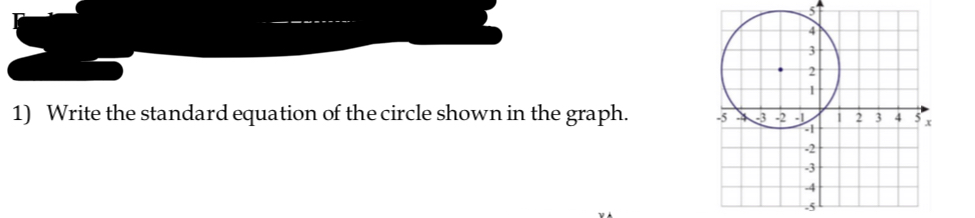# Write the standard equation of the circle shown in the graph.1)-53 -2-t.3

Question
41 viewshelp_outlineImage TranscriptioncloseWrite the standard equation of the circle shown in the graph. 1) -5 3 -2 -t .3 fullscreen
check_circle

Step 1

Known fact:

The standard equation of the circle is  (x - h)2 + (y -k)2 = r2

Step 2

Calculation:

In general, the fixed point and the fixed distance in a circle are said to be its centre and radius, respectively.

Thus, from the given graph, the centre  and the radius of the circle are observed to be (-2, 2) and 3, respecti...

### Want to see the full answer?

See Solution

#### Want to see this answer and more?

Solutions are written by subject experts who are available 24/7. Questions are typically answered within 1 hour.*

See Solution
*Response times may vary by subject and question.
Tagged in

### Algebra